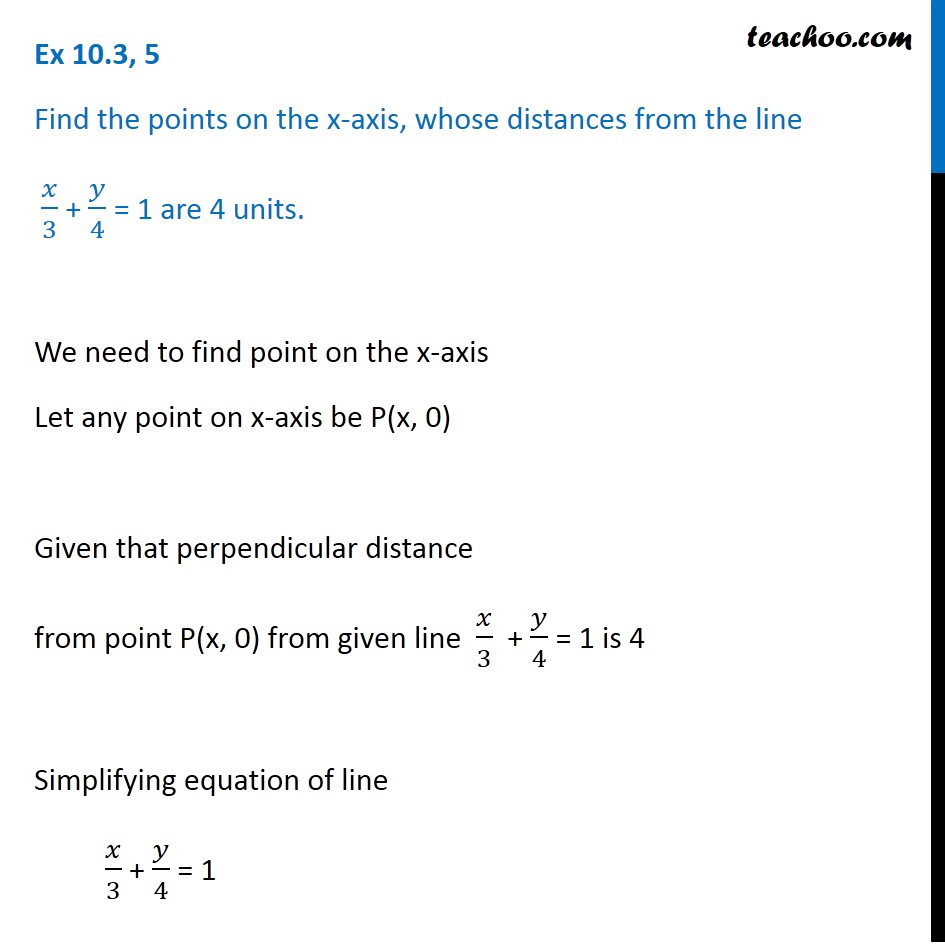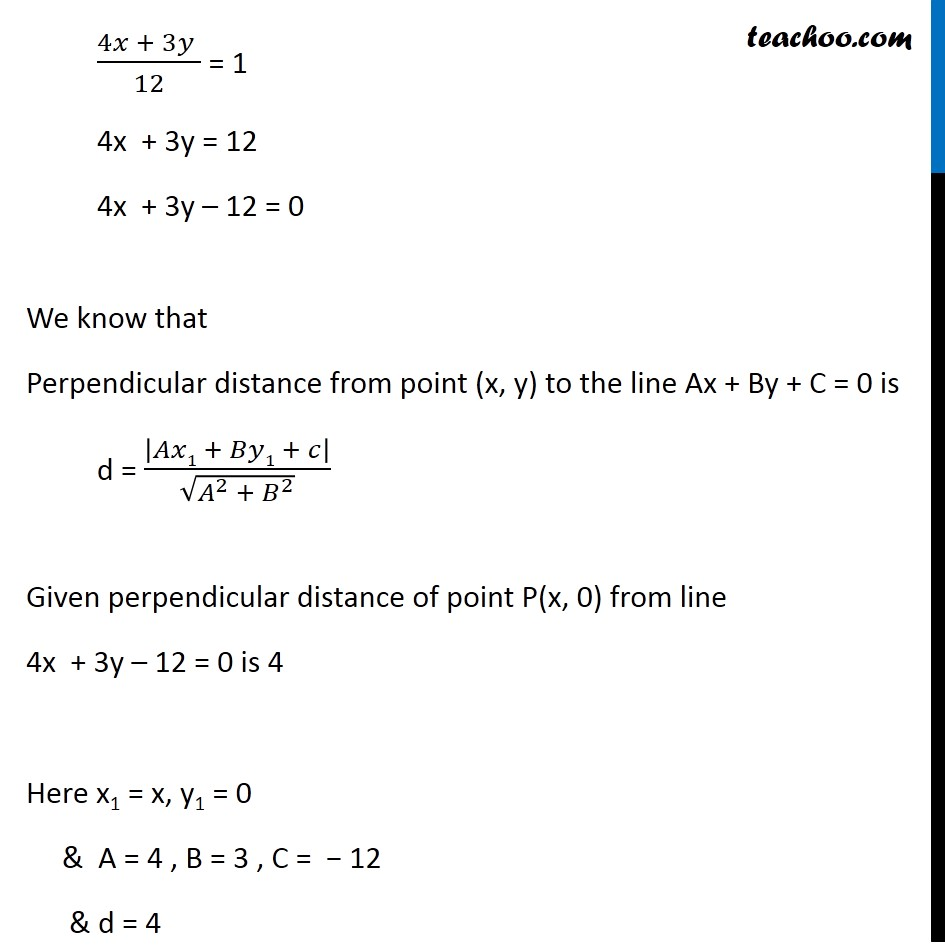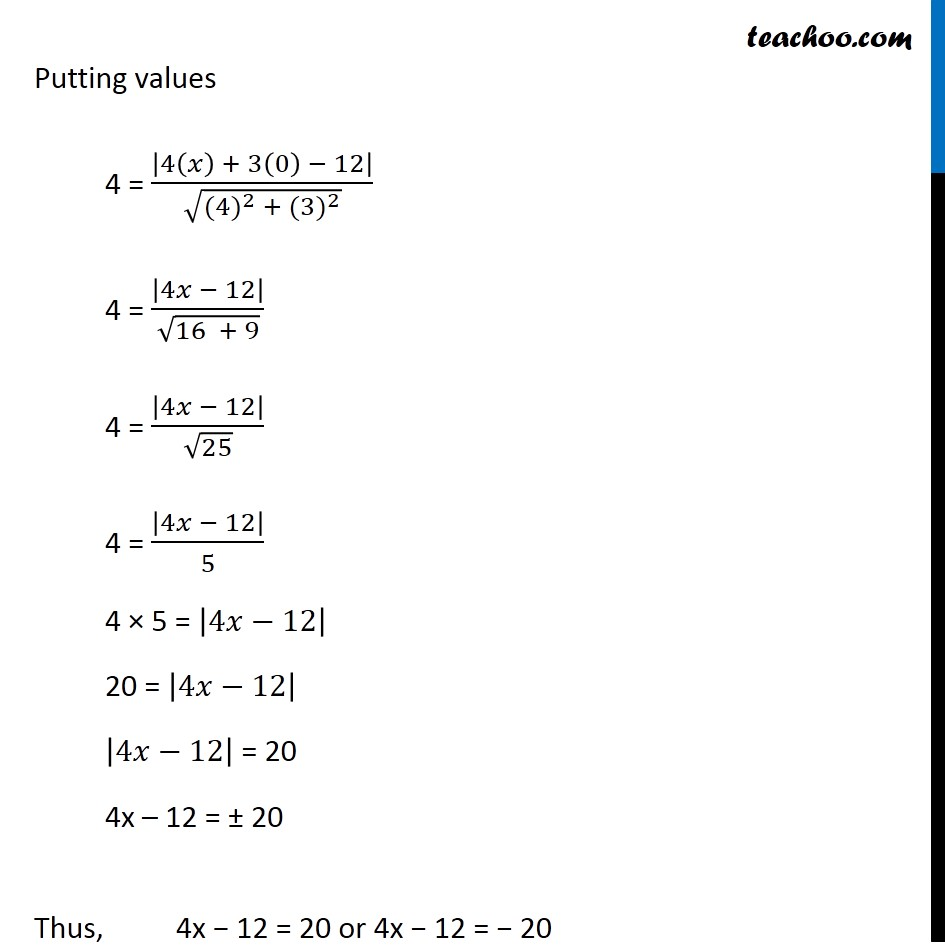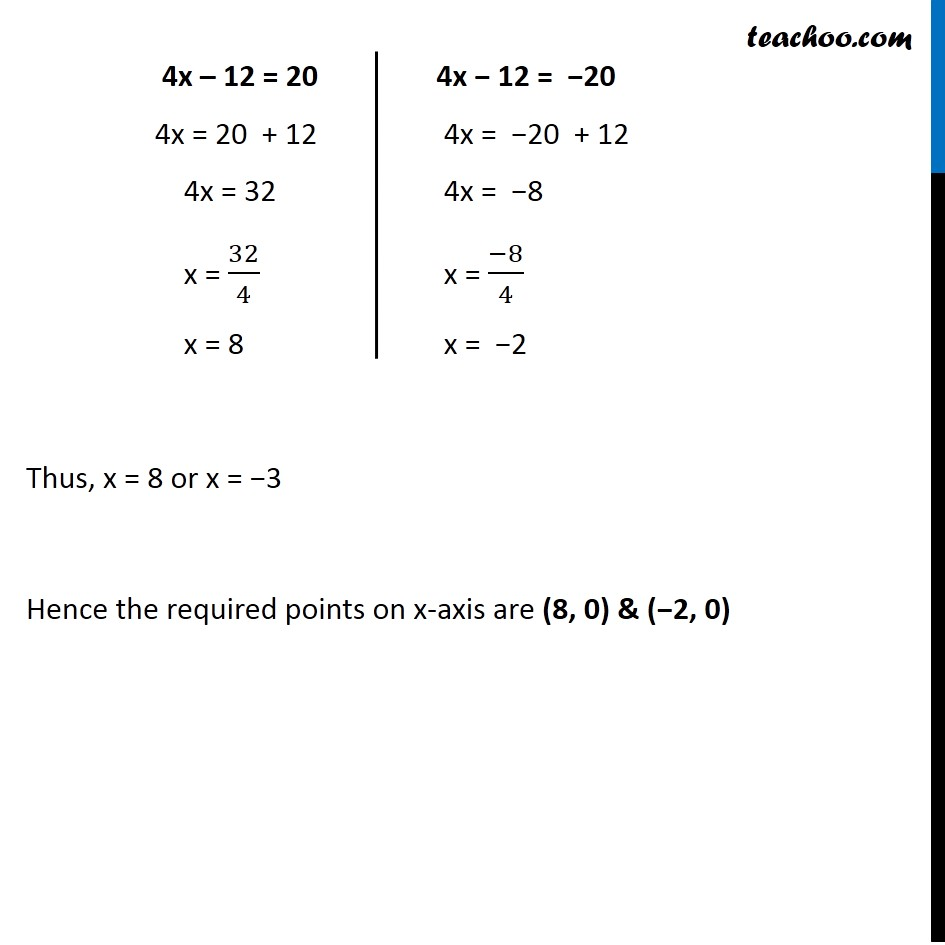Ex 9.3

Chapter 9 Class 11 Straight Lines
Serial order wiseLearn in your speed, with individual attention - Teachoo Maths 1-on-1 Class

### Transcript

Ex 9.3, 4 Find the points on the x-axis, whose distances from the line 𝑥/3 + 𝑦/4 = 1 are 4 units. We need to find point on the x-axis Let any point on x-axis be P(x, 0) Given that perpendicular distance from point P(x, 0) from given line 𝑥/3 + 𝑦/4 = 1 is 4 Simplifying equation of line 𝑥/3 + 𝑦/4 = 1 (4𝑥 + 3𝑦 )/12 = 1 4x + 3y = 12 4x + 3y – 12 = 0 We know that Perpendicular distance from point (x, y) to the line Ax + By + C = 0 is d = |𝐴𝑥1 + 𝐵𝑦1 + 𝑐|/√(𝐴^2 + 𝐵^2 ) Given perpendicular distance of point P(x, 0) from line 4x + 3y – 12 = 0 is 4 Here x1 = x, y1 = 0 & A = 4 , B = 3 , C = − 12 & d = 4 Putting values 4 = |4(𝑥) + 3(0) − 12|/√(〖(4)〗^2 + 〖(3)〗^2 ) 4 = |4𝑥 − 12|/√(16 + 9) 4 = |4𝑥 − 12|/√25 4 = |4𝑥 − 12|/5 4 × 5 = |4𝑥−12| 20 = |4𝑥−12| |4𝑥−12| = 20 4x – 12 = ± 20 Thus, 4x − 12 = 20 or 4x − 12 = − 20 4x – 12 = 20 4x = 20 + 12 4x = 32 x = 32/4 x = 8 4x − 12 = −20 4x = −20 + 12 4x = −8 x = (−8)/4 x = −2 4x − 12 = −20 4x = −20 + 12 4x = −8 x = (−8)/4 x = −2 Thus, x = 8 or x = −3 Hence the required points on x-axis are (8, 0) & (−2, 0)